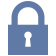Updated: 12/29/2021

# Statistical Measures

0%
Topic
Review Topic
0
0
N/A
N/A
Questions
2
0
0
0%
0%
Evidence
1
0
0
Topic
• Standard Deviation vs. Standard Error
• n = sample size
• Sigma (σ) = standard deviation
• SEM = standard error of the mean
• SEM = σ/√n
• SEM < σ
• SEM decreases as n increases
• z-scores
• 1 = +/- 1 σ around mean
• 2 = +/- 2 σ around mean
• 3 = +/- 3 σ around mean
• Confidence Interval (CI)
• Describes the range in which the mean would be expected to fall if the study were performed again and again
• = range from [mean - Z(SEM)] to [mean + Z(SEM)]
• 95% CI (alpha = 0.05) is standard
•  for 95% CI, Z = 1.96
• Outcomes
• if 0 falls within the CI when calculating the difference between 2 variables, H0 is not rejected and the result is not significantif
• 1 falls within the CI when calculating OR or RR, H0 is not rejected and the result is not significant
• T-test vs. ANOVA vs. χ2
• T-test
• compares the means of 2 groups on a continuous variable
•  ANOVA (analysis of variance)
• compares the means of 3 or more groups on a continuous variable
• χ2 ("chi-squared")
• tests whether 2 nominal variables are associated
• used with 2x2 tables
• e.g., effect of treatment on disease
• Correlation Coefficient (r)
• Pearson coefficient, r, is always between -1 and +1
• Absolute value indicates strength of correlation between 2 variables
• Coefficient of determination = r2
• Attributable Risk (AR)
• AR is incidence in the exposed (Ie) - incidence in the unexposed (Iu) = Ie - Iu
• Ie = a/(a+b)
• Iu = c/(c+d)
• AR = a/(a+b) - c/(c+d)
• The AR percent (ARP) is the attributable risk divided by incidence in the exposed (Ie)
• ARP = 100* (Ie-Iu)/Ie = 100*[a/(a+b) - c/(c+d)]/[a/(a+b)]
• note that relative risk (RR) = Ie/Iu = a/(a+b) DIVIDED BY c/(c+d)
• using math tricks
• ARP = (RR-1)/RR

Please rate this review topic.

You have never rated this topic.

Thank you. You can rate this topic again in 12 months.

Flashcards (0)
Cards
1 of 0
Questions (2)Sorry, this question is for
PEAK Premium Subscribers onlySorry, this question is for
PEAK Premium Subscribers only
Evidence (1)
VIDEOS & PODCASTS (1)